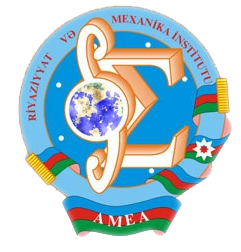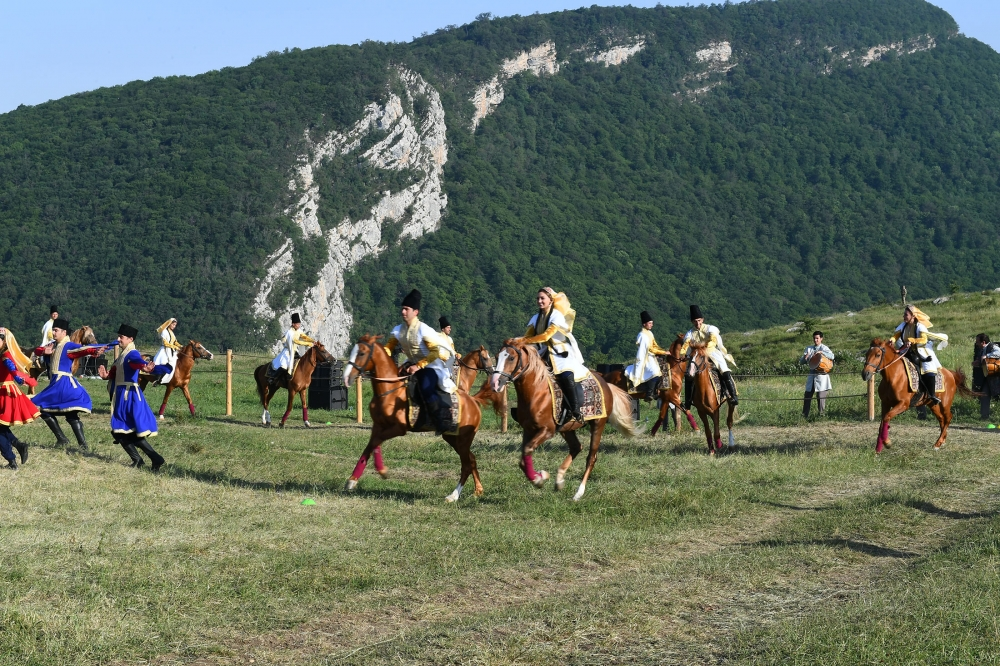Riyaziyyat və Mexanika İnstitutu

## Qarabağ xəbərləri### Şuşada Heydər Əliyev Fondunun təşkilatçılığı ilə “Musiqi irsi və Qarabağ atları Cıdır düzündə” adlı kompozisiya təqdim olunub## Transactions 2006/1/XXVI

 Year: 2006 Volume: 1 Number: XXVI MATHEMATICS Agayeva Ch.A. Abstract [PDF] A necessary condition for one stochastic optimal control problem with constant delay on control and state [PDF] 3 Aliyev A.B., Jabrailova G.D. Abstract [PDF] Quasilinear hyperbolic equations with discontinuous coefficients[PDF] 15 Aliyev Z.S. Abstract [PDF] Basis properties of the system of eigen functions on a fourth order problem with spectral and physical parameters in boundary condition [PDF] 25 Aslanova N.M. Abstract [PDF] Calculation of the regularized trace of differential operator with operator coefficient [PDF] 39 Bilalov B.T., Eminov M.S. Abstract [PDF] On completeness of a system of powers [PDF] 45 Gadjiev A.G., Aliyev S.A. Abstract [PDF] Galton-Watson branching processes with random errors[PDF] 51 Gadjiev T.S., Mamedova V.A. Abstract [PDF] Removable sets of solutions of the second boundary-value problem for second order parabolic equations [PDF] 59 Gasymov T.B. Abstract [PDF] On necessary and sufficient conditions of basicity of some defective systems in Banach spaces [PDF] 65 Guliyev E.V. Abstract [PDF] Weighted inequality for fractional maximal functions and fractional integrals, associated with the Laplace-Bessel differential operator [PDF] 71 Hajibayov M.G. Abstract [PDF] The potential-type integral operators on homogeneous groups[PDF] 81 Heydarov A.H. Abstract [PDF] Spectral analysis of one-dimensional biharmonic operator with 5”-potential [PDF] 89 Imanova M.N. Abstract [PDF] On one multistep method of numerical solution for the Volterra integral equation [PDF] 95 Jabbarova K.A. Abstract [PDF] Scattering problem for a system of five ordinary differential equations on a semi-axis[PDF] 105 Jafarov S.H. Abstract [PDF] Behavior of solution of a mixed boundary value problem for nonlinear elliptic equation at the neighborhood of a boundary point [PDF] 113 Kerimov N.B., Ibadov E.J. Abstract [PDF] On necessary conditions of basicity of a system of eigen-functions of second order discontinuous operators [PDF] 117 Mamedov F.I., Mirheydarli M.M. Abstract [PDF] On W2,p a priori estimation and uniqueness of solution of Neumann problem for quasilinear elliptic equations with discontinuous coefficients[PDF] 125 Mamedov Sh.H. Abstract [PDF] On equiconvergence rate of orthogonal expansion respondent to the fourth order differential operator [PDF] 137 Mammadova Sh.N. Abstract [PDF] Estimation of solutions of the first mixed problem for second order parabolic equations [PDF] 151 Ragimov F.G. Abstract [PDF] On the limit behavior of conditional probabilities of crossing of nonlinear boundaries by perturbed random walk[PDF] 163 MECHANICS Guliyev H.F., Mehdiyev A.A. Abstract [PDF] Application of l-moments problem to solution of one optimal control problem for the bar oscillation equation[PDF] 169 Mektiev M.F. Abstract [PDF] Construction of homogeneous solutions of a non-axially-symmetric tension problem of elasticity theory for transversely isotropic plates of variable thickness[PDF] 177 Talybly L.Kh., Agayev H.N. Abstract [PDF] Exact solution of a problem on deformation of physically nonlinear viscoelastic annuar plate of constant thickness at loading by external and internal pressures [PDF] 187 APPLIED PROBLEMS OF MATHEMATICS AND MECHANICS Akhmedov N.K. Abstract [PDF] Asymptotic analysis of torsion problem for a radially-inhomogeneous cylinder of small thickness [PDF] 193 Ibiev F.T., Sharifov Y.A. Abstract [PDF] An optimal control problem for Goursat systems with integral conditions[PDF] 199 Sadigov M.A. Abstract [PDF] On sufficient and necessary conditions of extremum with constraints[PDF] 207
Azərbaycanda COVID-19 ilə bağlı statistika
• Virusa yoluxan

599713

• Sağalan

569238

• Yeni yoluxan

1210

• Aktiv xəstə

22456

• Ölüm halı

8019

• Test edilib

5,633,054